Documentation

# 2-D Convolution

Compute 2-D discrete convolution of two input matrices

## Library

Filtering

`visionfilter`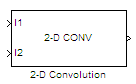## Description

The 2-D Convolution block computes the two-dimensional convolution of two input matrices. Assume that matrix A has dimensions (Ma, Na) and matrix B has dimensions (Mb, Nb). When the block calculates the full output size, the equation for the 2-D discrete convolution is

`$C\left(i,j\right)=\sum _{m=0}^{\left(Ma-1\right)}\sum _{n=0}^{\left(Na-1\right)}A\left(m,n\right)*B\left(i-m,j-n\right)$`

where $0\le i and $0\le j.

PortInput/OutputSupported Data TypesComplex Values Supported

I1

Matrix of intensity values or a matrix that represents one plane of the RGB video stream

• Double-precision floating point

• Single-precision floating point

• Fixed point

• 8-, 16-, 32-bit signed integer

• 8-, 16-, 32-bit unsigned integer

Yes

I2

Matrix of intensity values or a matrix that represents one plane of the RGB video stream

Same as I1 port

Yes

Output

Convolution of the input matrices

Same as I1 port

Yes

If the data type of the input is floating point, the output of the block has the same data type.

The dimensions of the output are dictated by the Output size parameter. Assume that the input at port I1 has dimensions (Ma, Na) and the input at port I2 has dimensions (Mb, Nb). If, for the Output size parameter, you choose `Full`, the output is the full two-dimensional convolution with dimensions (Ma+Mb-1, Na+Nb-1). If, for the Output size parameter, you choose `Same as input port I1`, the output is the central part of the convolution with the same dimensions as the input at port I1. If, for the Output size parameter, you choose `Valid`, the output is only those parts of the convolution that are computed without the zero-padded edges of any input. This output has dimensions (Ma-Mb+1, Na-Nb+1). However, if `all(size(I1)<size(I2))`, the block errors out.

If you select the Normalized output check box, the block's output is divided by `sqrt(sum(dot(I1p,I1p))*sum(dot(I2,I2)))`, where `I1p` is the portion of the I1 matrix that aligns with the I2 matrix. See Example 2 for more information.

### Note

When you select the Normalized output check box, the block input cannot be fixed point.

### Fixed-Point Data Types

The following diagram shows the data types used in the 2-D Convolution block for fixed-point signals.You can set the product output, accumulator, and output data types in the block mask as discussed in Parameters.

The output of the multiplier is in the product output data type if at least one of the inputs to the multiplier is real. If both of the inputs to the multiplier are complex, the result of the multiplication is in the accumulator data type. For details on the complex multiplication performed, refer to Multiplication Data Types.

## Examples

### Example 1

Suppose I1, the first input matrix, has dimensions (4,3) and I2, the second input matrix, has dimensions (2,2). If, for the Output size parameter, you choose `Full`, the block uses the following equations to determine the number of rows and columns of the output matrix:

``
`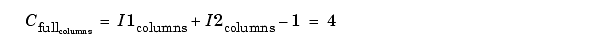`

The resulting matrix is

``

If, for the Output size parameter, you choose ```Same as input port I1```, the output is the central part of $Cfull$ with the same dimensions as the input at port I1, (4,3). However, since a 4-by-3 matrix cannot be extracted from the exact center of $Cfull$, the block leaves more rows and columns on the top and left side of the $Cfull$ matrix and outputs:

``

If, for the Output size parameter, you choose `Valid`, the block uses the following equations to determine the number of rows and columns of the output matrix: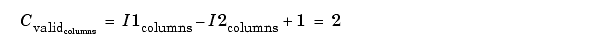In this case, it is always possible to extract the exact center of $Cfull$. Therefore, the block outputs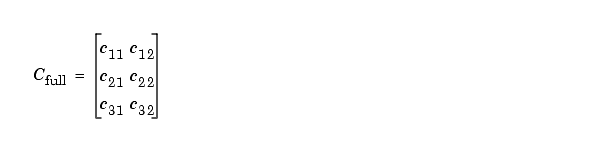### Example 2

In convolution, the value of an output element is computed as a weighted sum of neighboring elements.

For example, suppose the first input matrix represents an image and is defined as

```I1 = [17 24 1 8 15 23 5 7 14 16 4 6 13 20 22 10 12 19 21 3 11 18 25 2 9] ```

The second input matrix also represents an image and is defined as

```I2 = [8 1 6 3 5 7 4 9 2] ```

The following figure shows how to compute the (1,1) output element (zero-based indexing) using these steps:

1. Rotate the second input matrix, I2, 180 degrees about its center element.

2. Slide the center element of I2 so that it lies on top of the (0,0) element of I1.

3. Multiply each element of the rotated I2 matrix by the element of I1 underneath.

4. Sum the individual products from step 3.

Hence the (1,1) output element is $0\cdot 2+0\cdot 9+0\cdot 4+0\cdot 7+17\cdot 5+24\cdot 3+0\cdot 6+23\cdot 1+5\cdot 8=220$.

Computing the (1,1) Output of Convolution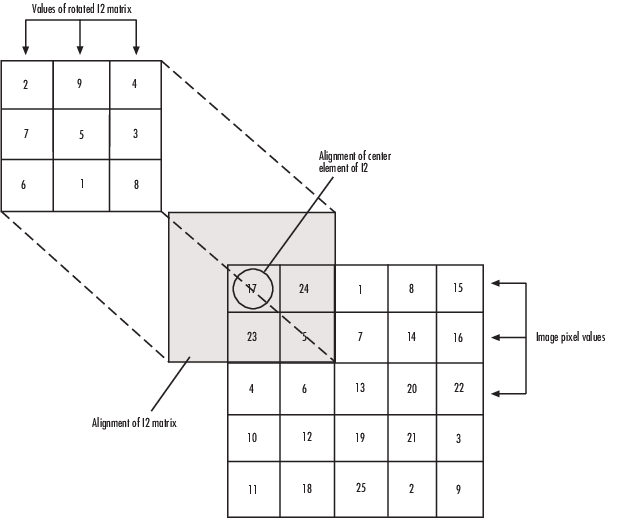The normalized convolution of the (1,1) output element is `220/sqrt(sum(dot(I1p,I1p))*sum(dot(I2,I2))) `= 0.3459, where ```I1p = [0 0 0; 0 17 24; 0 23 5]```.

## Parameters

Output size

This parameter controls the size of the output scalar, vector, or matrix produced as a result of the convolution between the two inputs. If you choose `Full`, the output has dimensions (Ma+Mb-1, Na+Nb-1). If you choose ```Same as input port I1```, the output has the same dimensions as the input at port I1. If you choose `Valid`, output has dimensions (Ma-Mb+1, Na-Nb+1).

Normalized output

If you select this check box, the block's output is normalized.

Rounding mode

Select the rounding mode for fixed-point operations.

Overflow mode

Select the Overflow mode for fixed-point operations.

Product output

Use this parameter to specify how to designate the product output word and fraction lengths. Refer to Fixed-Point Data Types and Multiplication Data Types for illustrations depicting the use of the product output data type in this block:

• When you select `Same as first input`, these characteristics match those of the first input to the block.

• When you select `Binary point scaling`, you can enter the word length and the fraction length of the product output, in bits.

• When you select `Slope and bias scaling`, you can enter the word length, in bits, and the slope of the product output. The bias of all signals in the Computer Vision Toolbox™ software is 0.

The Product Output inherits its sign according to the inputs. If either or both input I1 and I2 are signed, the Product Output will be signed. Otherwise, the Product Output is unsigned. The following table shows all cases.

Sign of Input I1Sign of Input I2Sign of Product Output
unsignedunsignedunsigned
unsignedsignedsigned
signedunsignedsigned
signedsignedsigned

Accumulator

Use this parameter to specify how to designate the accumulator word and fraction lengths. Refer to Fixed-Point Data Types and Multiplication Data Types for illustrations depicting the use of the accumulator data type in this block. The accumulator data type is only used when both inputs to the multiplier are complex:

• When you select `Same as product output`, these characteristics match those of the product output.

• When you select `Same as first input`, these characteristics match those of the first input to the block.

• When you select `Binary point scaling`, you can enter the word length and the fraction length of the accumulator, in bits.

• When you select `Slope and bias scaling`, you can enter the word length, in bits, and the slope of the accumulator. The bias of all signals in the Computer Vision Toolbox software is 0.

Output

Choose how to specify the word length and fraction length of the output of the block:

• When you select `Same as first input`, these characteristics match those of the first input to the block.

• When you select `Binary point scaling`, you can enter the word length and the fraction length of the output, in bits.

• When you select `Slope and bias scaling`, you can enter the word length, in bits, and the slope of the output. The bias of all signals in the Computer Vision Toolbox software is 0.

Lock data type settings against change by the fixed-point tools

Select this parameter to prevent the fixed-point tools from overriding the data types you specify on the block mask. For more information, see `fxptdlg`, a reference page on the Fixed-Point Tool in the Simulink® documentation.

## See Also

 2-D FIR Filter Computer Vision Toolbox software

Download ebook